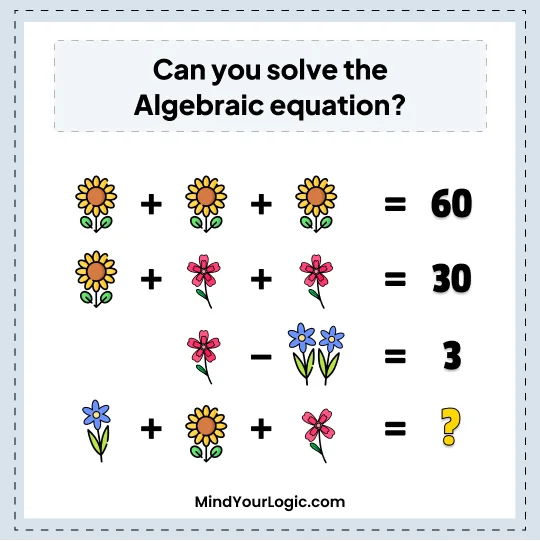# Algebraic equation - Math Riddle

Can you solve the Algebraic equation?Explanation :

First Equation
1Red Flower + 1Red Flower + 1Red Flower = 60
Hence, 1Red Flower = 20
Second Equation
Red Flower + 5Blue Petals + 5Blue Petals = 30
20 + 10Blue Petals = 30
10Blue Petals = 10
Hence 1Blue Petals = 1
Third Equation
5Blue Petals + 2Yellow Flower = 3
5 + 2Yellow Flower = 3
2Yellow Flower = 2
Hence, 1Yellow Flower = 1
Required
Yellow Flower + Red Flower + 4Blue Petals = ?
Hence, 1 + 20 + 4 = 25